Main Content

# comm.MIMOChannel

Filter input signal through MIMO multipath fading channel

## Description

A comm.MIMOChannel object filters an input signal through a multiple-input/multiple-output (MIMO) multipath fading channel. This object models both Rayleigh and Rician fading and employs the Kronecker model for modeling the spatial correlation between the links. For processing details, see the Algorithms section.

To filter an input signal through a MIMO multipath fading channel:

1. Create the `comm.MIMOChannel` object and set its properties.

2. Call the object with arguments, as if it were a function.

To learn more about how System objects work, see What Are System Objects?.

## Creation

### Syntax

``mimochan = comm.MIMOChannel``
``mimochan = comm.MIMOChannel(Name,Value)``

### Description

````mimochan = comm.MIMOChannel` creates a multiple-input multiple-output (MIMO) frequency-selective or frequency-flat fading channel System object™. This object filters a real or complex input signal through the multipath MIMO channel to obtain the channel-impaired signal.```

example

````mimochan = comm.MIMOChannel(Name,Value)` sets properties using one or more name-value pairs. Enclose each property name in single quotes.Example: `comm.MIMOChannel('SampleRate',2)````

## Properties

expand all

Unless otherwise indicated, properties are nontunable, which means you cannot change their values after calling the object. Objects lock when you call them, and the `release` function unlocks them.

If a property is tunable, you can change its value at any time.

For more information on changing property values, see System Design in MATLAB Using System Objects.

Input signal sample rate in hertz, specified as a positive scalar.

Data Types: `double`

Discrete path delay in seconds, specified as a scalar or row vector.

• When you set `PathDelays` to a scalar, the MIMO channel is frequency flat.

• When you set `PathDelays` to a vector, the MIMO channel is frequency selective.

Data Types: `double`

Average path gains in decibels, specified as a scalar or row vector. `AveragePathGains` must have the same size as PathDelays.

Data Types: `double`

Normalize path gains, specified as `true` or `false`.

• When you set this property to `true`, the fading processes are normalized so that the total power of the path gains, averaged over time, is `0` dB.

• When you set this property to `false`, there is no normalization on path gains.

The average powers of the path gains are specified by the AveragePathGains property.

Data Types: `logical`

Fading distribution to use for the channel, specified as `'Rayleigh'` or `'Rician'`.

Data Types: `char`

K-factor of a Rician fading channel, specified as a positive scalar or a 1-by-NP vector of positive-valued elements. NP equals number of path delays specified by the PathDelays property.

• If you set `KFactor` to a scalar, the first discrete path is a Rician fading process with a Rician K-factor of `KFactor`. Any remaining discrete paths are independent Rayleigh fading processes.

• If you set `KFactor` to a row vector, the discrete path corresponding to a positive element of the `KFactor` vector is a Rician fading process with a Rician K-factor specified by that element. The discrete path corresponding to a zero-valued element of the `KFactor` vector is a Rayleigh fading process.

#### Dependencies

This property applies when FadingDistribution is `Rician`.

Data Types: `double`

Doppler shifts for the line-of-sight components of the Rician fading channel in hertz, specified as a scalar or row vector. This property must have the same size as KFactor.

• If you set `DirectPathDopplerShift` to a scalar, it represents the line-of-sight component Doppler shift of the first discrete path that is a Rician fading process.

• If you set `DirectPathDopplerShift` to a row vector, the discrete path that is a Rician fading process has its line-of-sight component Doppler shift specified by the elements of `DirectPathDopplerShift` that correspond to positive elements in the KFactor vector.

#### Dependencies

This property applies when FadingDistribution is `Rician`.

Data Types: `double`

Initial phases for the line-of-sight components of the Rician fading channel in radians, specified as a scalar or row vector. This property must have the same size as KFactor.

• If you set `DirectPathInitialPhase` to a scalar, it represents the line-of-sight component initial phase of the first discrete path that is a Rician fading process.

• If you set `DirectPathInitialPhase` to a row vector, the discrete path that is a Rician fading process has its line-of-sight component initial phase specified by the elements of `DirectPathInitialPhase` that correspond to positive elements in the KFactor vector.

#### Dependencies

This property applies when FadingDistribution is `Rician`.

Data Types: `double`

Maximum Doppler shift for all channel paths in hertz, specified as a nonnegative scalar.

The Doppler shift applies to all channel paths. When you set this property to `0`, the channel remains static for the entire input. You can use the `reset` object function to generate a new channel realization.

`MaximumDopplerShift` must be smaller than (SampleRate/10)/fc for each path, where fc represents the cutoff frequency factor of the path. For more information on the cutoff frequency, see Cutoff Frequency Factor.

Data Types: `double`

Doppler spectrum shape for all channel paths, specified as a single Doppler spectrum structure returned from the `doppler` function or a 1-by-NP cell array of such structures. The default value of this property is the Jakes Doppler spectrum (`doppler('Jakes')`).

• If you assign a single call to `doppler`, all paths have the same specified Doppler spectrum.

• If you assign a 1-by-NP cell array of calls to `doppler` using any of the specified syntaxes, each path has the Doppler spectrum specified by the corresponding Doppler spectrum structure in the array. In this case, NP equals the value of the PathDelays property.

The maximum Doppler shift value necessary to specify the Doppler spectrum/spectra is given by the MaximumDopplerShift property.

#### Dependencies

This property applies when MaximumDopplerShift is greater than zero.

If you assign the FadingTechnique property to ```'Sum of sinusoids'```, you must set `DopplerSpectrum` to `doppler('Jakes')`.

Spatial correlation specification, specified as ```'Separate Tx Rx'```, `'None'`, or `'Combined'`.

• Choose `'Spatial Tx Rx'` to separately specify the transmit and receive spatial correlation matrices from which the number of transmit antenna (NT) and number of receive antennas (NR) are derived.

• Choose `'None'` to specify the number of transmit and receive antennas.

• Choose `'Combined'` to specify a single correlation matrix for the whole channel, from which the product of NT and NR is derived.

Data Types: `char`

Number of transmit antennas, specified as a positive integer.

#### Dependencies

This property applies when SpatialCorrelationSpecification is `'None'` or `'Combined'`.

Data Types: `double`

Number of receive antennas, specified as a positive integer.

#### Dependencies

This property applies when SpatialCorrelationSpecification is `'None'` or `'Combined'`.

Data Types: `double`

Specify the spatial correlation of the transmitter as an NT-by-NT matrix or NT-by-NT-by-NP array. NT is the number of transmit antennas, and NP equals the value of the PathDelays property.

• If `PathDelays` is a scalar, the channel is frequency-flat, and `TransmitCorrelationMatrix` is an NT-by-NT Hermitian matrix. The magnitude of any off-diagonal element must be no larger than the geometric mean of the two corresponding diagonal elements.

• If `PathDelays` is a vector, the channel is frequency selective, and you can specify `TransmitCorrelationMatrix` as a matrix. Each path has the same transmit spatial correlation matrix.

• Alternatively, you can specify `TransmitCorrelationMatrix` as an NT-by-NT-by-NP array, where each path can have its own different transmit spatial correlation matrix.

#### Dependencies

This property applies when you set the SpatialCorrelationSpecification property to `'Separate Tx Rx'`.

Data Types: `double`
Complex Number Support: Yes

Specify the spatial correlation of the receiver as an NR-by-NR matrix or NR-by-NR-by-NP array. NR is the number of receive antennas, and NP equals the value of the PathDelays property.

• If PathDelays is a scalar, the channel is frequency flat, and `ReceiveCorrelationMatrix` is an NR-by-NR Hermitian matrix. The magnitude of any off-diagonal element must be no larger than the geometric mean of the two corresponding diagonal elements.

• If PathDelays is a vector, the channel is frequency selective, and you can specify `ReceiveCorrelationMatrix` as a matrix. Each path has the same receive spatial correlation matrix.

• Alternatively, you can specify `ReceiveCorrelationMatrix` as an NR-by-NR-by-NP array, where each path can have its own different receive spatial correlation matrix.

#### Dependencies

This property applies when you set the SpatialCorrelationSpecification property to `'Separate Tx Rx'`.

Data Types: `double`
Complex Number Support: Yes

Combined spatial correlation matrix, specified as an NTR-by-NTR matrix or NTR-by-NTR-by-NP array, where NTR = (NTNR), and NP equals the value of the PathDelays property.

• If PathDelays is a scalar, the channel is frequency flat, and `SpatialCorrelationMatrix` is an NTR-by-NTR Hermitian matrix. The magnitude of any off-diagonal element must be no larger than the geometric mean of the two corresponding diagonal elements.

• If PathDelays is a vector, the channel is frequency selective, and you can specify `SpatialCorrelationMatrix` as a matrix. Each path has the same spatial correlation matrix.

• Alternatively, you can specify `SpatialCorrelationMatrix` as an NTR-by-NTR-by-NP array, where each path can have its own different combined spatial correlation matrix.

#### Dependencies

This property applies when you set the SpatialCorrelationSpecification property to `'Combined'`.

Data Types: `double`
Complex Number Support: Yes

Antenna selection scheme, specified as `'Off'`, `'Tx'`, `'Rx'`, or ```'Tx and Rx'```.

`Tx` represents transmit antennas and `Rx` represents receive antennas. When you configure any antenna selection other than the default setting, the object requires one or more inputs to specify which antennas are selected for signal transmission. For more information, see Antenna Selection.

Data Types: `char`

Normalize channel outputs, specified as `true` or `false`.

• When you set this property to `true`, channel outputs are normalized by the number of receive antennas.

• When you set this property to `false`, channel outputs are not normalized.

Data Types: `logical`

Channel model fading technique, specified as ```'Filtered Gaussian noise'``` or `'Sum of sinusoids'`.

Data Types: `char`

Number of sinusoids used to model the fading process, specified as a positive integer.

#### Dependencies

This property applies when FadingTechnique is ```'Sum of sinusoids'```.

Data Types: `double`

Source to control the start time of the fading process, specified as `'Property'` or `'Input port'`.

• `'Property'` -- Use the InitialTime property to set the initial time offset.

• `'Input port'` -- Specify the start time of the fading process by using the `initialtime` input to the object. The input value can change in consecutive calls to the object.

#### Dependencies

This property applies when FadingTechnique is ```'Sum of sinusoids'```.

Initial time offset for the fading model in seconds, specified as a nonnegative scalar.

When `InitialTime` is not a multiple of 1/SampleRate, it is rounded up to the nearest sample position.

#### Dependencies

This property applies when the FadingTechnique property is set to ```'Sum of sinusoids'``` and the InitialTimeSource property is set to `'Property'`.

Data Types: `double`

Source of the random number stream, specified as ```'Global stream'``` or `'mt19937ar with seed'`.

• `'Global stream'` -- The current global random number stream is used for normally distributed random number generation. In this case, the `reset` object function resets the filters only.

• `'mt19937ar with seed'` -- The mt19937ar algorithm is used for normally distributed random number generation. In this case, the `reset` object function resets the filters and also reinitializes the random number stream to the value of the Seed property.

Data Types: `char`

Initial seed of the mt19937ar random number stream, specified as a nonnegative integer. When the `reset` object function is called, the mt19937ar random number stream is reinitialized to the `Seed` value.

#### Dependencies

This property applies when you set the RandomStream property to ```'mt19937ar with seed'```.

Data Types: `double`

Option to output path gains, specified as `false` or `true`. Set this property to `true` to output the channel path gains of the underlying fading process.

Data Types: `logical`

Channel visualization preference, specified as `'Off'`, `'Impulse response'`, ```'Frequency response'```, ```'Impulse and frequency responses'```, or `'Doppler spectrum'`. When visualization is on, the selected channel characteristics, such as impulse response or Doppler spectrum, display in a separate window. For more information, see Channel Visualization.

#### Dependencies

Visualization applies only when the FadingTechnique property is set to ```'Filtered Gaussian noise'```.

Transmit-receive antenna pair to display, specified as a 1-by-2 vector, where the first element corresponds to the desired transmit antenna and the second element corresponds to the desired receive antenna. At this time, only a single pair can be displayed.

#### Dependencies

This property applies when Visualization is not `Off`.

Path for which the Doppler spectrum is displayed, specified as a positive integer from 1 to NP, where NP equals the value of the PathDelays property.

#### Dependencies

This property applies when Visualization is set to ```'Doppler spectrum'```.

Percentage of samples to display, specified as `10%`, `25%`, `50%`, or `100%`. Increasing the percentage improves display accuracy at the expense of simulation speed.

#### Dependencies

This property applies when Visualization is `'Impulse response'`, `'Frequency response'`, or ```'Impulse and frequency responses'```.

## Usage

### Syntax

``outsignal = mimochan(insignal)``
``outsignal = mimochan(insignal,seltx)``
``outsignal = mimochan(insignal,selrx)``
``outsignal = mimochan(insignal,seltx,selrx)``
``outsignal = mimochan(___,initialtime)``
``[outsignal,pathgains] = mimochan(___)``

### Description

example

````outsignal = mimochan(insignal)` filters the input signal through the MIMO fading channel specified by `mimochan` and returns the result in `outsignal`.```
````outsignal = mimochan(insignal,seltx)` turns on the transmit antennas selected by `seltx` for channel processing.This syntax applies when you set the AntennaSelection property of the object to `'Tx'`.For example, to select the first and third transmit antenna index as active: mimochan = comm.MIMOChannel('AntennaSelection','Tx'); seltx = [1 0 1]; ... outsignal = mimochan(insignal,seltx);```
````outsignal = mimochan(insignal,selrx)` turns on receive antennas, selected by `selrx` for channel processing. This syntax applies when you set the AntennaSelection property of the object to `'Rx'`.For example, to select the second receive antenna index as active: mimochan = comm.MIMOChannel('AntennaSelection','Rx'); selrx = [0 1]; ... outsignal = mimochan(insignal,selrx);```

example

````outsignal = mimochan(insignal,seltx,selrx)` turns on transmit and receive antennas, selected by `seltx` and `selrx` for channel processing. This syntax applies when you set the AntennaSelection property of the object to `'Tx and Rx'`.For example: mimochan = comm.MIMOChannel('AntennaSelection','Tx and Rx'); seltx = [1 1]; selrx = [0 1]; ... outsignal = mimochan(insignal,selrx);```

example

````outsignal = mimochan(___,initialtime)` specifies a start time for the fading process. This syntax applies when you set the FadingTechnique property of the object to `'Sum of sinusoids'` and the InitialTimeSource property of the object to ```'Input port'```. The syntax supports input options from prior syntaxes.```

example

````[outsignal,pathgains] = mimochan(___)` also returns the MIMO channel path gains for antenna selection schemes. The syntax supports input options from prior syntaxes.```

### Input Arguments

expand all

Input signal, specified as a scalar, an NS element column vector, an NS-by-NT matrix, or an NS-by-NST matrix.

• NS is the number of samples.

• NT is the number of transmit antennas. NT is determined by the TransmitCorrelationMatrix or NumTransmitAntennas property values of the object.

• NST is the number of selected transmit antennas, as determined by the number of elements set to `1` in the vector provided to the seltx input.

The number of transmit antennas is determined by the TransmitCorrelationMatrix or NumTransmitAntennas property values of the object.

Data Types: `double` | `single`
Complex Number Support: Yes

Select active transmit antennas, specified as a 1-by-NT binary vector. NT represents the number of transmit antennas. Elements set to `1` identify selected antenna indices and `0` identify nonselected antenna indices.

Data Types: `double`

Select active receive antennas, specified as a 1-by-NR binary vector. NR represents the number of receive antennas. Elements set to `1` identify selected antenna indices and `0` identify nonselected antenna indices.

Data Types: `double`

Initial time offset for the fading model in seconds, specified as a nonnegative scalar.

The initial time offset must be greater than the last frame end time. When `initialtime` is not a multiple of 1/SampleRate, it is rounded up to the nearest sample position.

Data Types: `double`

### Output Arguments

expand all

Output data signal, returned as an NS-by-NR or NS-by-NSR matrix.

• NS is the number of samples.

• NR is the number of receive antennas. NR is determined by the ReceiveCorrelationMatrix or NumReceiveAntennas property values of the object.

• NSR is the number of selected receive antennas, as determined by the number of elements set to `1` in the vector provided to the selrx input.

Output path gains, returned as an NS-by-NP-by-NT-by-NR array with `NaN` values for the unselected transmit-receive antenna pairs.

• NS is the number of samples.

• NP equals the value of the PathDelays property.

• NT is the number of transmit antennas.

• NR is the number of receive antennas.

## Object Functions

To use an object function, specify the System object as the first input argument. For example, to release system resources of a System object named `obj`, use this syntax:

`release(obj)`

expand all

 `info` Characteristic information about fading channel object
 `step` Run System object algorithm `release` Release resources and allow changes to System object property values and input characteristics `reset` Reset internal states of System object

Note

• If you set the `RandomStream` property of the object to ```'Global stream'```, the `reset` object function resets the filters only.

• If you set `RandomStream` to `'mt19937ar with seed'`, the `reset` object function resets the filters and also reinitializes the random number stream to the value of the `Seed` property.

## Examples

collapse all

Create a 4-by-2 MIMO channel by using the MIMO channel System object. Modulate and spatially encode data, and then pass the data through the channel.

Generate QPSK-modulated data.

```data = randi([0 3],1000,1); modData = pskmod(data,4,pi/4);```

Create an orthogonal space-time block encoder to encode the modulated data into four spatially separated streams. Encode the data.

```ostbc = comm.OSTBCEncoder('NumTransmitAntennas',4,'SymbolRate',1/2); txSig = ostbc(modData);```

Create a MIMO channel object, using name-value pairs to set the properties. The channel consists of two paths with a maximum Doppler shift of 5 Hz. Set the `SpatialCorrelationSpecification` property to `'None'`, which requires that you specify the number of transmit and receive antennas. Set the number of transmit antennas to 4 and the number of receive antennas to 2.

```mimochannel = comm.MIMOChannel('SampleRate',1000, ... 'PathDelays',[0 2e-3],'AveragePathGains',[0 -5], ... 'MaximumDopplerShift',5,'SpatialCorrelationSpecification','None', ... 'NumTransmitAntennas',4,'NumReceiveAntennas',2);```

Pass the modulated and encoded data through the MIMO channel.

`rxSig = mimochannel(txSig);`

Create a time vector, `t`, to use for plotting the power of the received signal.

```ts = 1/mimochannel.SampleRate; t = (0:ts:(size(txSig,1)-1)*ts)';```

Calculate and plot the power of the signal received by antenna 1.

```pwrdB = 20*log10(abs(rxSig(:,1))); plot(t,pwrdB) xlabel('Time (s)') ylabel('Power (dBW)')```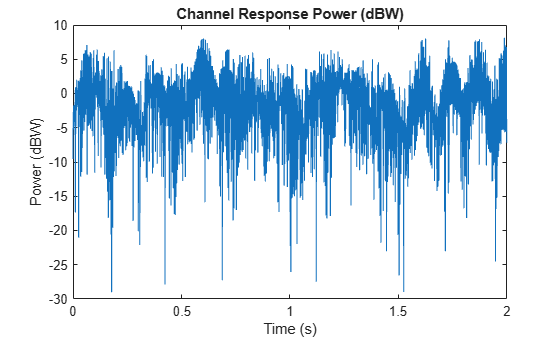Without specifying antenna selection, filter PSK-modulated data through a 2-by-2 Rayleigh fading channel and examine the spatial correlation characteristics of the channel realization. Use the `release` object function to unlock the object to set the `AntennaSelection` property to `'Tx and Rx'` and then confirm the unselected transmit-receive antenna pairs.

Examine Spatial Correlation Characteristics Without Specifying Antenna Selection

Create a PSK modulator System object™ to modulate randomly generated data.

```pskModulator = comm.PSKModulator; modData = pskModulator(randi([0 pskModulator.ModulationOrder-1],1e5,1));```

Split the modulated data into two spatial streams.

`channelInput = reshape(modData,[2 5e4]).';`

Create a 2-by-2 MIMO channel System object with two discrete paths. Each path has different transmit and receive correlation matrices, specified by the `TransmitCorrelationMatrix` and `ReceiveCorrelationMatrix` properties.

```mimoChan = comm.MIMOChannel('SampleRate',1000, 'PathDelays',[0 1e-3], ... 'AveragePathGains',[3 5], 'NormalizePathGains',false, 'MaximumDopplerShift',5, ... 'TransmitCorrelationMatrix',cat(3,eye(2),[1 0.1;0.1 1]), ... 'ReceiveCorrelationMatrix',cat(3,[1 0.2;0.2 1],eye(2)), ... 'RandomStream','mt19937ar with seed', 'Seed',33, 'PathGainsOutputPort',true);```

Filter the modulated data using the MIMO channel object.

`[~,pathGains] = mimoChan(channelInput);`

The transmit spatial correlation for the first discrete path at the first receive antenna is specified as an identity matrix in the `TransmitCorrelationMatrix` property. Confirm that the channel output `pathGains` exhibits the same statistical characteristics by using the `corrcoef` function.

`disp('Tx spatial correlation, first path, first Rx:');`
```Tx spatial correlation, first path, first Rx: ```
`disp(corrcoef(squeeze(pathGains(:,1,:,1))));`
``` 1.0000 + 0.0000i 0.0357 - 0.0253i 0.0357 + 0.0253i 1.0000 + 0.0000i ```

The transmit spatial correlation for the second discrete path at the second receive antenna is specified as `[1 0.1;0.1 1]` in the `TransmitCorrelationMatrix` property. Confirm that the channel output `pathGains` exhibits the same statistical characteristics.

`disp('Tx spatial correlation, second path, second Rx:');`
```Tx spatial correlation, second path, second Rx: ```
`disp(corrcoef(squeeze(pathGains(:,2,:,2))));`
``` 1.0000 + 0.0000i 0.0863 + 0.0009i 0.0863 - 0.0009i 1.0000 + 0.0000i ```

The receive spatial correlation for the first discrete path at the second transmit antenna is specified as `[1 0.2;0.2 1]` in the `ReceiveCorrelationMatrix` property. Confirm that the channel output `pathGains` exhibits the same statistical characteristics.

`disp('Rx spatial correlation, first path, second Tx:');`
```Rx spatial correlation, first path, second Tx: ```
`disp(corrcoef(squeeze(pathGains(:,1,2,:))));`
``` 1.0000 + 0.0000i 0.2236 + 0.0550i 0.2236 - 0.0550i 1.0000 + 0.0000i ```

The receive spatial correlation for the second discrete path at the first transmit antenna is specified as an identity matrix in the `ReceiveCorrelationMatrix` property. Confirm that the channel output `pathGains` exhibits the same statistical characteristics.

`disp('Rx spatial correlation, second path, first Tx:');`
```Rx spatial correlation, second path, first Tx: ```
`disp(corrcoef(squeeze(pathGains(:,2,1,:))));`
``` 1.0000 + 0.0000i -0.0088 - 0.0489i -0.0088 + 0.0489i 1.0000 + 0.0000i ```

Examine Spatial Correlation Characteristics Specifying Antenna Selection

Enable transmit and receive antenna selection for the `mimoChan` object. The input frame size is shortened to 100.

```release(mimoChan); mimoChan.AntennaSelection = 'Tx and Rx'; modData = pskModulator(randi([0 pskModulator.ModulationOrder-1],100,1));```

Select the first transmit antenna and second receive antenna.

`[channelOutput,pathGains] = mimoChan(modData,[1 0],[0 1]);`

Confirm that the path gains that MATLAB® returns have `NaN` values for the unselected transmit-receive antenna pairs.

`disp('Return 1 if the path gains for the second transmit antenna are NaN:');`
```Return 1 if the path gains for the second transmit antenna are NaN: ```
`disp(isequal(isnan(squeeze(pathGains(:,:,2,:))), ones(100,2,2)));`
``` 1 ```
`disp('Return 1 if the path gains for the first receive antenna are NaN:');`
```Return 1 if the path gains for the first receive antenna are NaN: ```
`disp(isequal(isnan(squeeze(pathGains(:,:,:,1))), ones(100,2,2)));`
``` 1 ```

Create a frequency selective MIMO channel and display its impulse and frequency responses.

Set the sample rate to 10 MHz and specify path delays and gains using the extended vehicular A (EVA) channel parameters. Set the maximum Doppler shift to 70 Hz.

```fs = 10e6; % Hz pathDelays = [0 30 150 310 370 710 1090 1730 2510]*1e-9; % sec avgPathGains = [0 -1.5 -1.4 -3.6 -0.6 -9.1 -7 -12 -16.9]; % dB fD = 70; % Hz ```

Create a 2x2 MIMO channel System object with the previously defined parameters and set the `Visualization` property to `Impulse and frequency responses` using name-value pairs. By default, the antenna pair corresponding to transmit antenna 1 and receive antenna 1 will be displayed.

```mimoChan = comm.MIMOChannel('SampleRate',fs, ... 'PathDelays',pathDelays, ... 'AveragePathGains',avgPathGains, ... 'MaximumDopplerShift',fD, ... 'Visualization','Impulse and frequency responses'); ```

Generate random binary data and pass it through the MIMO channel. The impulse response plot allows you to easily identify the individual paths and their corresponding filter coefficients. The frequency selective nature of the EVA channel is shown by the frequency response plot.

```x = randi([0 1],1000,2); y = mimoChan(x); ```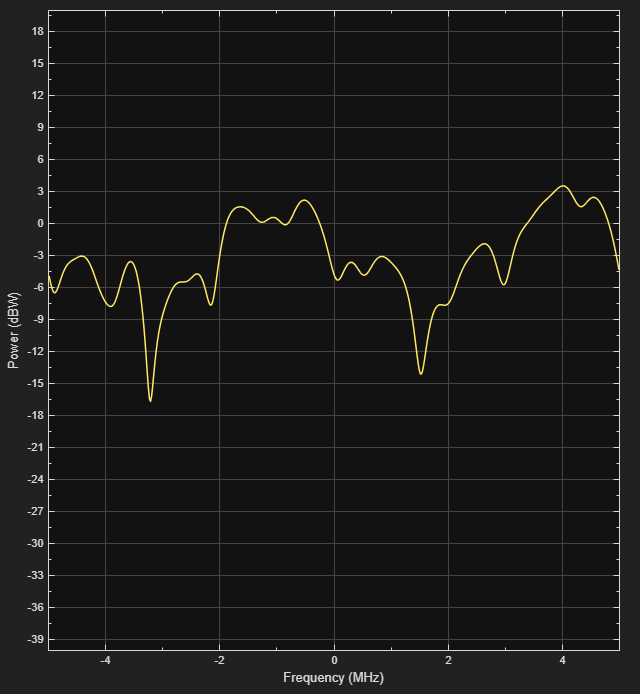Release `mimoChan` and set the `AntennaPairsToDisplay` property to [2 1] to view the antenna pair corresponding to transmit antenna 2 and receive antenna 1. It is necessary to release the object as the property is non-tunable.

```release(mimoChan) mimoChan.AntennaPairsToDisplay = [2 1]; y = mimoChan(x); ```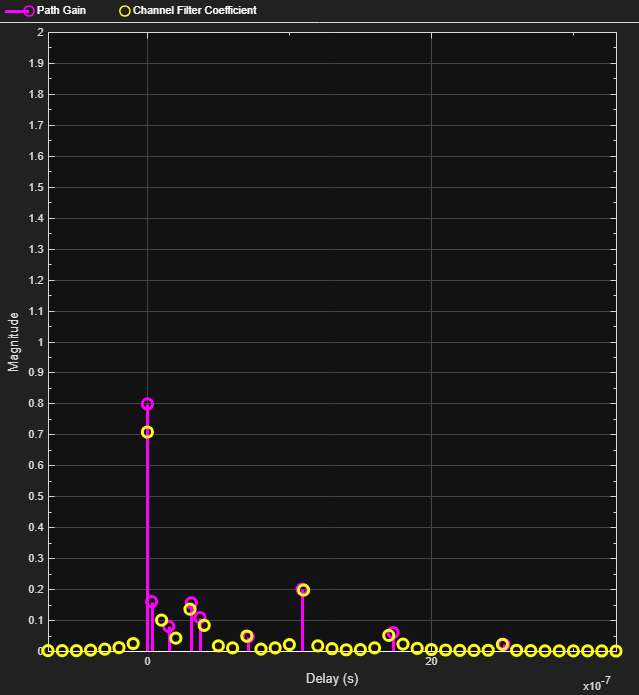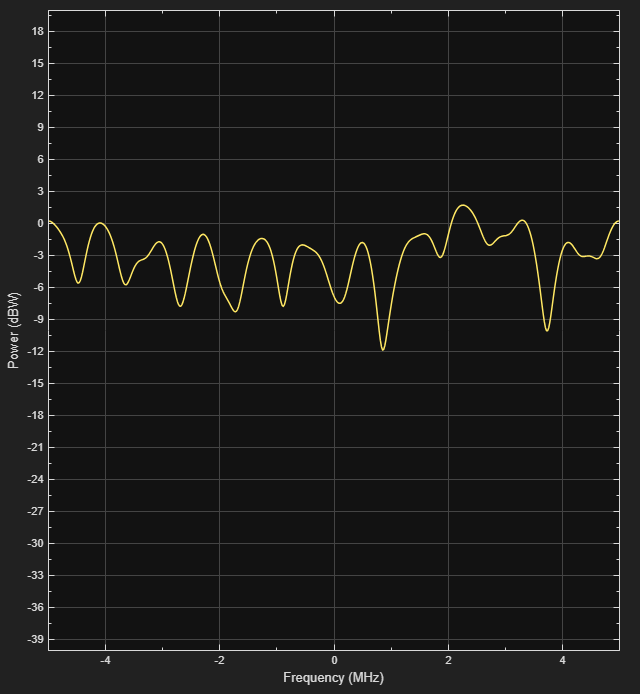Create and visualize the Doppler spectra of a MIMO channel having two paths.

Construct a cell array of Doppler structures to be used in creating the channel. The Doppler spectrum of the first path is set to have a bell shape while the second path is set to be flat.

```dp{1} = doppler('Bell'); dp{2} = doppler('Flat'); ```

Create a default 2x2 MIMO channel with two paths and a 100 Hz maximum Doppler shift using name-value pairs. Set the `Visualization` property to `Doppler spectrum` and set `PathsForDopplerDisplay` to 1. The Doppler spectrum of the first path will be displayed.

```mimoChan = comm.MIMOChannel('SampleRate',1000, ... 'PathDelays',[0 0.002], ... 'AveragePathGains',[0 -3], ... 'MaximumDopplerShift',100, ... 'DopplerSpectrum',dp, ... 'Visualization','Doppler spectrum', ... 'PathsForDopplerDisplay',1); ```

Pass random data through the MIMO channel to generate the Doppler spectrum of the first path. Since the Doppler spectrum plot only updates when its buffer is filled, the `mimoChan` function is invoked multiple times to improve the accuracy of the estimate. Observe that the spectrum has a bell shape and that its minimum and maximum frequencies fall within the limits set by `MaximumDopplerShift`.

```for k = 1:25 x = randi([0 1],10000,2); y = mimoChan(x); end ```Release `mimoChan` and set the `PathsForDopplerDisplay` property to 2. It is necessary to release the object as the property is non-tunable. Call the function multiple times to display the Doppler spectrum of the second path. Observe that the spectrum is flat.

```release(mimoChan) mimoChan.PathsForDopplerDisplay = 2; for k = 1:25 x = randi([0 1],10000,2); y = mimoChan(x); end ```Create a MIMO channel object and pass data through it using the sum-of-sinusoids technique. The example demonstrates how the channel state is maintained in cases in which data is discontinuously transmitted.

Define the overall simulation time and three time segments for which data will be transmitted. In this case, the channel is simulated for 1 s with a 1000 Hz sampling rate. One 1000-sample, continuous data sequence is transmitted at time 0. Three 100-sample data packets are transmitted at time 0.1 s, 0.4 s, and 0.7 s.

```t0 = 0:0.001:0.999; % Transmission 0 t1 = 0.1:0.001:0.199; % Transmission 1 t2 = 0.4:0.001:0.499; % Transmission 2 t3 = 0.7:0.001:0.799; % Transmission 3```

Generate random binary data corresponding to the previously defined time intervals.

```d0 = randi([0 1],1000,2); % 1000 samples d1 = randi([0 1],100,2); % 100 samples d2 = randi([0 1],100,2); % 100 samples d3 = randi([0 1],100,2); % 100 samples```

Create a flat fading 2x2 MIMO channel System object with the `Sum of sinusoids` fading technique. So that results can be repeated, specify a seed using a name-value pair. As the `InitialTime` property is not specified, the fading channel will be simulated from time 0. Enable the path gains output port.

```mimoChan1 = comm.MIMOChannel('SampleRate',1000, ... 'MaximumDopplerShift',5, ... 'RandomStream','mt19937ar with seed', ... 'Seed',17, ... 'FadingTechnique','Sum of sinusoids', ... 'PathGainsOutputPort',true);```

Create a clone of the MIMO channel System object. Set the `InitialTimeSource` property to `Input port` so that the fading channel offset time can be specified as an input argument to the `mimoChan` function.

```mimoChan2 = clone(mimoChan1); mimoChan2.InitialTimeSource = 'Input port';```

Pass random binary data through the first channel object, `mimoChan1`. Data is transmitted over all 1000 time samples. For this example, only the complex path gain is needed.

`[~,pg0] = mimoChan1(d0);`

Pass random data through the second channel object, `mimoChan2`, where the initial time offsets are provided as input arguments.

```[~,pg1] = mimoChan2(d1,0.1); [~,pg2] = mimoChan2(d2,0.4); [~,pg3] = mimoChan2(d3,0.7);```

Compare the number of samples processed by the two channels using the `info` method. You can see that 1000 samples were processed by `mimoChan1` while only 300 were processed by `mimoChan2`.

```G = info(mimoChan1); H = info(mimoChan2); [G.NumSamplesProcessed H.NumSamplesProcessed]```
```ans = 1×2 1000 300 ```

Convert the path gains into decibels for the path corresponding to the first transmit and first receive antenna.

```pathGain0 = 20*log10(abs(pg0(:,1,1,1))); pathGain1 = 20*log10(abs(pg1(:,1,1,1))); pathGain2 = 20*log10(abs(pg2(:,1,1,1))); pathGain3 = 20*log10(abs(pg3(:,1,1,1)));```

Plot the path gains for the continuous and discontinuous cases. Observe that the gains for the three segments perfectly match the gain for the continuous case. The alignment of the two highlights that the sum-of-sinusoids technique is ideally suited to the simulation of packetized data as the channel characteristics are maintained even when data is not transmitted.

```plot(t0,pathGain0,'r--') hold on plot(t1,pathGain1,'b') plot(t2,pathGain2,'b') plot(t3,pathGain3,'b') grid xlabel('Time (sec)') ylabel('Path Gain (dB)') legend('Continuous','Discontinuous','location','nw')```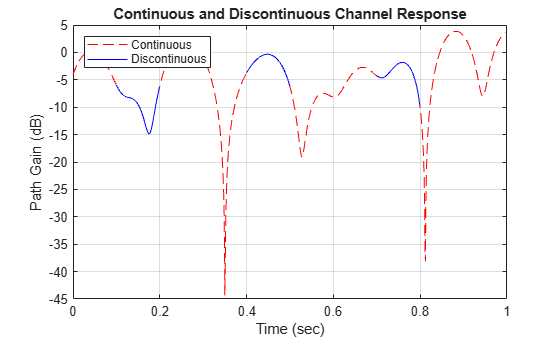Demonstrate the advantage of using the sum of sinusoids fading technique when simulating a channel with burst data.

Set the simulation parameters such that the sampling rate is 100 kHz, the total simulation time is 100 seconds, and the duty cycle for the burst data is 25%.

```fs = 1e5; % Hz tsim = 100; % seconds dutyCycle = 0.25;```

Create a flat fading 2x2 MIMO channel object using the default `Filtered Gaussian noise` technique.

`fgn = comm.MIMOChannel('SampleRate',fs);`

Create a similar MIMO channel object using the `Sum of sinusoids` technique where the fading process start times are given as an input argument.

```sos = comm.MIMOChannel('SampleRate',fs, ... 'FadingTechnique','Sum of sinusoids', ... 'NumSinusoids',48, ... 'InitialTimeSource','Input port');```

Run a continuous sequence of random bits through the filtered Gaussian noise MIMO channel object. Use the tic/toc stopwatch timer functions to measure the execution time of the function call.

```tic y = fgn(randi([0 1],fs*tsim,2)); tFGN = toc;```

To transmit a data burst each second, pass random bits through the sum of sinusoids MIMO channel object by calling the `sos` function inside of a for loop. Use the tic/toc stopwatch timer to measure the execution time.

```tic for k = 1:tsim z = sos(randi([0 1],fs*dutyCycle,2),0.5+(k-1)); end tSOS = toc;```

Compare the ratio of the sum of sinusoids execution time to the filtered Gaussian noise execution time. The ratio is less than one, which indicates that the sum of sinusoids technique is faster.

`tSOS/tFGN`
```ans = 0.2634 ```

## Algorithms

expand all

The fading processing per link is described in Methodology for Simulating Multipath Fading Channels and assumes the same parameters for all (NT × NR) links of the MIMO channel. Each link comprises all multipaths for that link.

 Oestges, C., and B. Clerckx. MIMO Wireless Communications: From Real-World Propagation to Space-Time Code Design, Academic Press, 2007.

 Correira, L. M. Mobile Broadband Multimedia Networks: Techniques, Models and Tools for 4G, Academic Press, 2006.

 Kermoal, J. P., L. Schumacher, K. I. Pedersen, P. E. Mogensen, and F. Frederiksen. "A stochastic MIMO radio channel model with experimental validation." IEEE Journal on Selected Areas of Communications. Vol. 20, Number 6, 2002, pp. 1211–1226.

 Jeruchim, M., P. Balaban, and K. S. Shanmugan. Simulation of Communication Systems, Second Edition, New York: Kluwer Academic/Plenum, 2000.

 Pätzold, Matthias, Cheng-Xiang Wang, and Bjorn Olav Hogstand. "Two New Sum-of-Sinusoids-Based Methods for the Efficient Generation of Multiple Uncorrelated Rayleigh Fading Waveforms." IEEE Transactions on Wireless Communications. Vol. 8, Number 6, 2009, pp. 3122–3131.

## Support

#### Bridging Wireless Communications Design and Testing with MATLAB

Download white paper